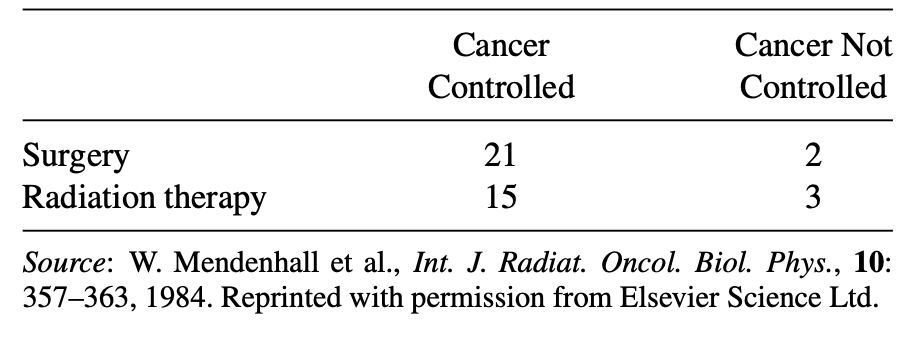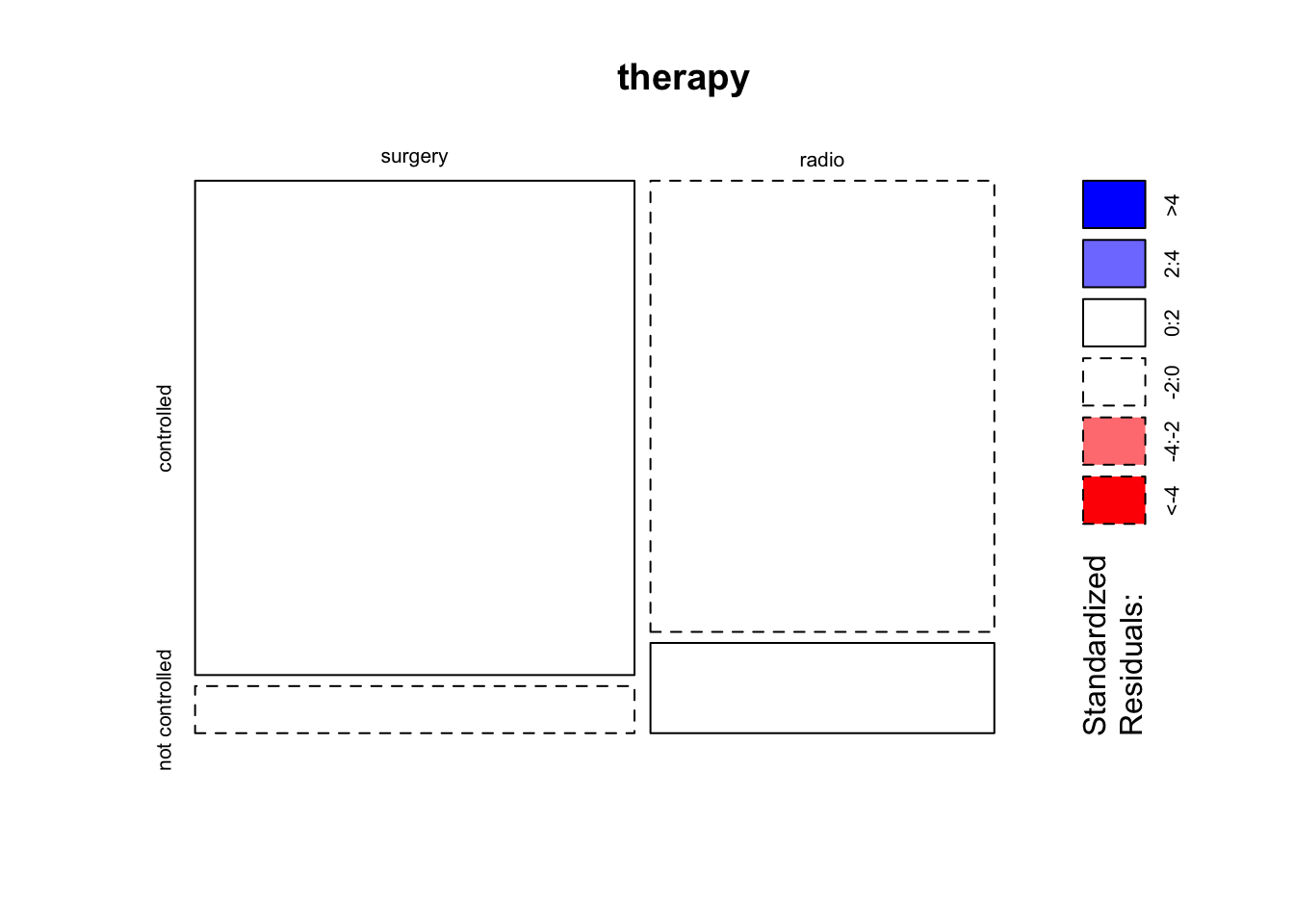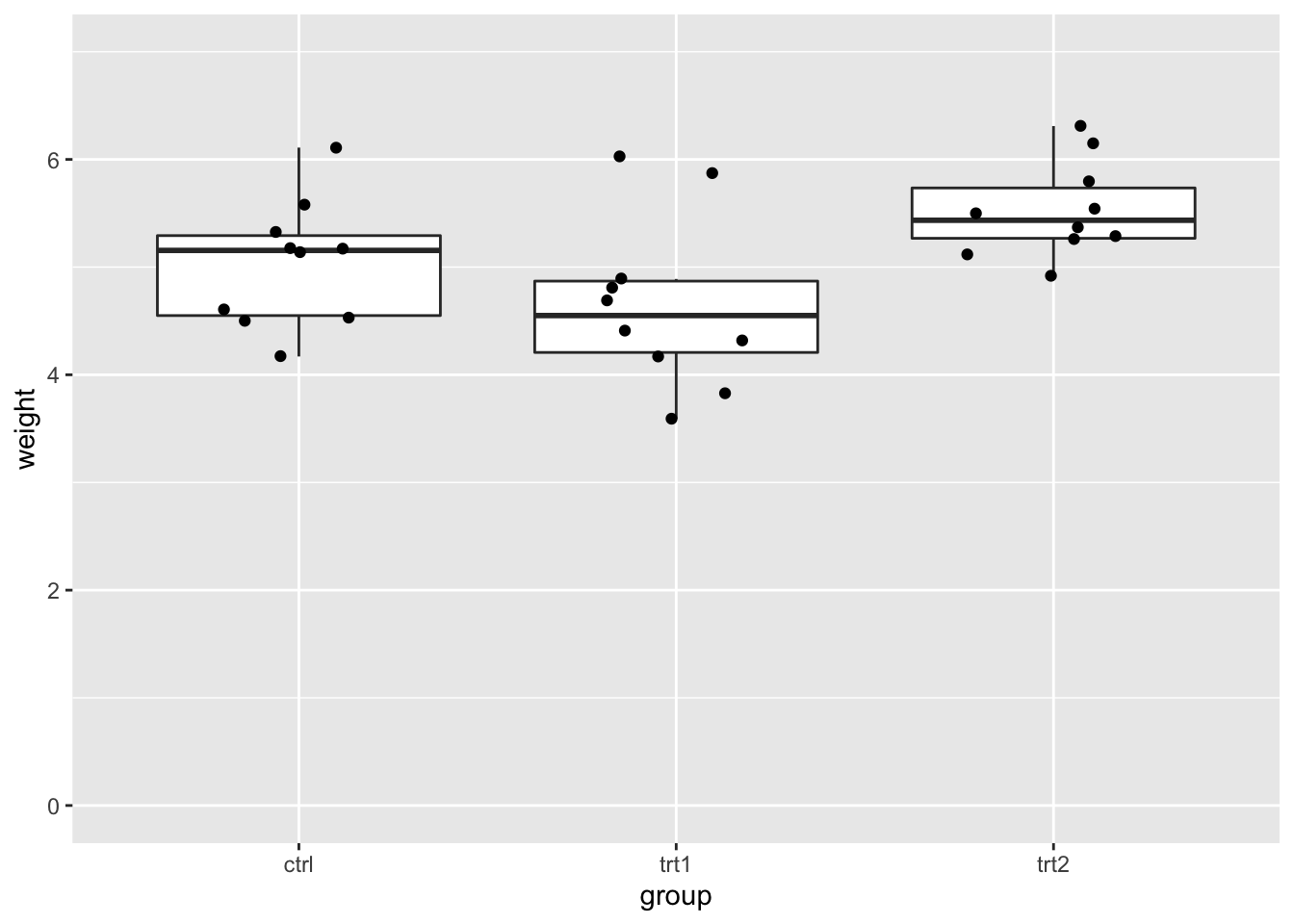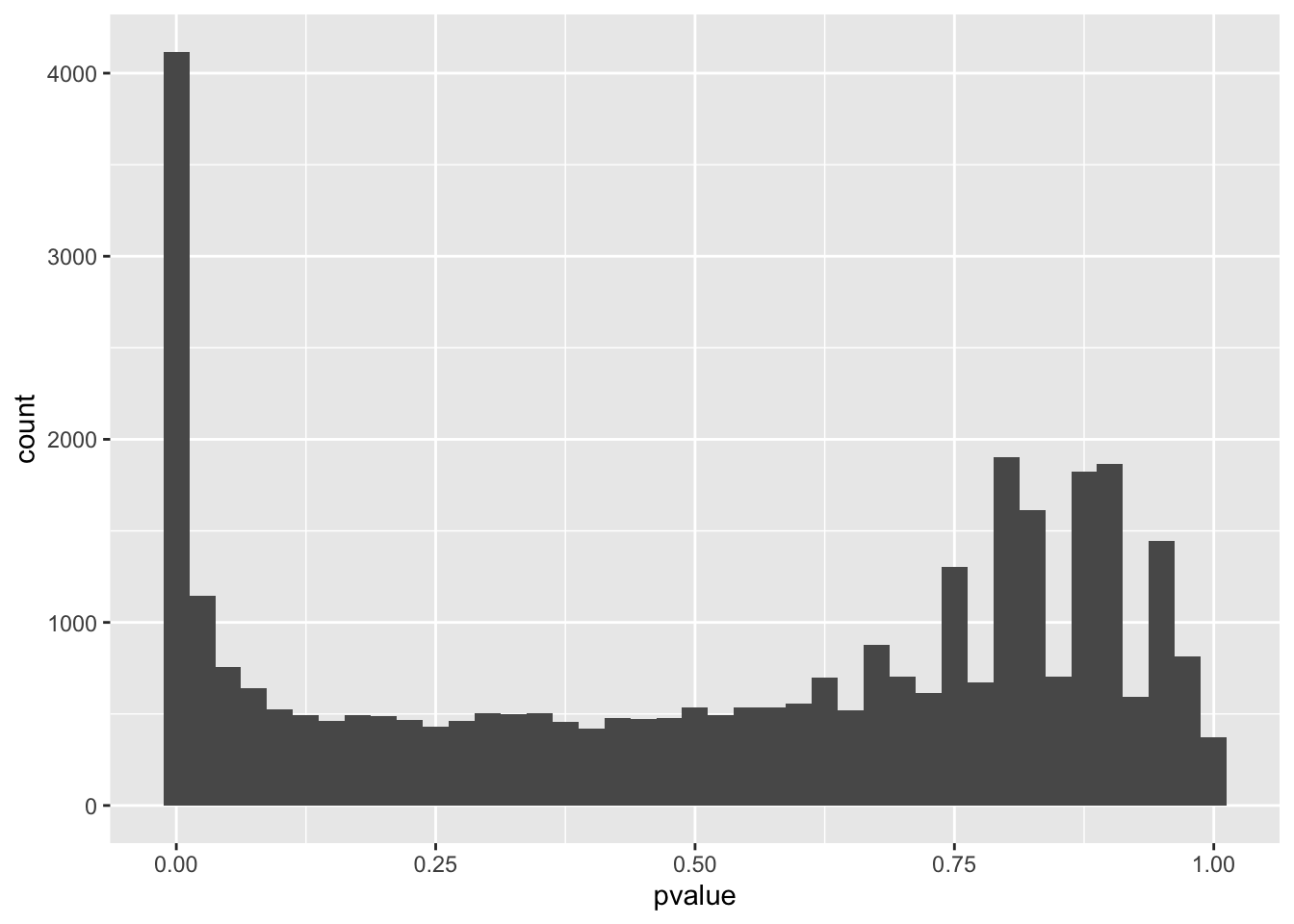Required packages:

library(tidyverse)
library(DESeq2)
library(airway)

# Chi square testTable taken from .

We can transform these study reports into a 2x2 matrix:

therapy <- matrix(c(21,15,2,3), nrow=2)
colnames(therapy) = c("controlled", "not controlled")
therapy
##         controlled not controlled
## surgery         21              2
## radio           15              3

Then perform a chi-square test:

chisq.test(therapy)
## Warning in chisq.test(therapy): Chi-squared approximation may be incorrect
##
##  Pearson's Chi-squared test with Yates' continuity correction
##
## data:  therapy
## X-squared = 0.085967, df = 1, p-value = 0.7694

R gives a warning, because in tables with low counts (<5) it is usually advised to use the Fisher-test instead of the chi-square test. The null and alternative hypotheses are the same in both cases, but the technical details differ.

fisher.test(therapy)
##
##  Fisher's Exact Test for Count Data
##
## data:  therapy
## p-value = 0.6384
## alternative hypothesis: true odds ratio is not equal to 1
## 95 percent confidence interval:
##   0.2089115 27.5538747
## sample estimates:
## odds ratio
##   2.061731

For visualization, we can use the mosaic plot:

mosaicplot(therapy, shade=TRUE)# Plant growth data

In the PlantGrowth data, two treatments and a control condition were tested with respect to the yield, i.e. the resulting weights of the plants.

As always, we first look at the data:

ggplot(PlantGrowth, aes(x=group, y=weight))+
geom_boxplot(outlier.alpha=0)+
geom_jitter(width=0.25)+
scale_y_continuous(limits=c(0,7))To test whether any of the groups differ from each other, we can use the ANOVA F-test. It tests against the null hypothesis that all three groups are equal.

my_aov <- aov(lm(weight ~ group, data=PlantGrowth))
my_aov
## Call:
##    aov(formula = lm(weight ~ group, data = PlantGrowth))
##
## Terms:
##                    group Residuals
## Sum of Squares   3.76634  10.49209
## Deg. of Freedom        2        27
##
## Residual standard error: 0.6233746
## Estimated effects may be unbalanced
summary(my_aov)
##             Df Sum Sq Mean Sq F value Pr(>F)
## group        2  3.766  1.8832   4.846 0.0159 *
## Residuals   27 10.492  0.3886
## ---
## Signif. codes:  0 '***' 0.001 '**' 0.01 '*' 0.05 '.' 0.1 ' ' 1

To explain the code: The ANOVA is performed on a linear model object, which we define with the lm function. Here, we set up a linear model that models the plant weight as a function of the treatment group.

The ANOVA is significant, which means not all groups are equal. We can then perform a follow-up Tukey test, to obtain adjusted p-values for the individual comparisons:

TukeyHSD(my_aov)
##   Tukey multiple comparisons of means
##     95% family-wise confidence level
##
## Fit: aov(formula = lm(weight ~ group, data = PlantGrowth))
##
## $group ## diff lwr upr p adj ## trt1-ctrl -0.371 -1.0622161 0.3202161 0.3908711 ## trt2-ctrl 0.494 -0.1972161 1.1852161 0.1979960 ## trt2-trt1 0.865 0.1737839 1.5562161 0.0120064 Three t-tests with Bonferroni correction: control <- filter(PlantGrowth, group=="ctrl") %>% select(weight) trt1 <- filter(PlantGrowth, group=="trt1") %>% select(weight) trt2 <- filter(PlantGrowth, group=="trt2") %>% select(weight) p_vals <- c(t.test(control,trt1)$p.value,
t.test(control,trt2)$p.value, t.test(trt1,trt2)$p.value)

p_vals
##  0.250382509 0.047899256 0.009298405
p.adjust(p_vals,method="bonferroni")
##  0.75114753 0.14369777 0.02789521

These two procedures (ANOVA followed by TukeyHSD and Bonferroni-corrected t-tests) test against the same null hypothesis: “All groups are equal.” But they differ technically, resulting in slightly different adjusted p-values.

# P-value adjustment for expression data

This in an example from Chapter 6 in MSMB.

data("airway")
aw   = DESeqDataSet(se = airway, design = ~ cell + dex)
aw   = DESeq(aw)
## estimating size factors
## estimating dispersions
## gene-wise dispersion estimates
## mean-dispersion relationship
## final dispersion estimates
## fitting model and testing
awde = as.data.frame(results(aw)) %>% dplyr::filter(!is.na(pvalue))

The above commands load the airway data and run a differential expression analysis on them. This data set contains read counts per gene for airway smooth muscle cell lines in an RNA-Seq experiment. The aim of this experiment was to compare the treatment with dex (dexamethasone, a drug which is used to treat asthma) to a control (no treatment). Another variable that differs between the samples is the cell line, encoded by cell. DESeq2 runs a test for every gene to check whether the dex treatment has a significant effect on its expression. The design ~cell + dex specifies a model where the read count is modeled as the sum of the cell line and a treatment effect. The residuals are modeled as gamma-poisson distributed.

We can make a histogram of the p-values:

awde %>%
ggplot(aes(pvalue))+
geom_histogram(binwidth=0.025)Usually, we control for the false discovery rate in high-throughput experiments. This is done with the Benjamini-Hochberg algorithm:

adjusted_pvalues <- p.adjust(awde$pvalue, method="BH") Let’s compare the number of DE genes found with un-adjusted and adjusted p-values: # unadjusted with alpha = 0.5 sum(awde$pvalue < 0.05)
##  5677
# adkusted with FDR = 0.1
sum(adjusted_pvalues < 0.05)
##  3467